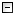Home Control Panel Login Register Search Submit Logout About me Contact meMy ResearchWeb This Site
 Home > Using OpenBUGS with SAS
Using OpenBUGS with SAS
2009-08-01    Zhang, Z.       Read: 18296 times
Cite this page: Zhang, Z. (2009). Using OpenBUGS with SAS. Retrieved December 6, 2023, from https://www.psychstat.org/us/article.php/84.htm.
 Using OpenBUGS with SAS Please cite this using Zhang, Z., McArdle, J. J., Wang, L., & Hamagami, F. (2008). A SAS interface for Bayesian analysis with WinBUGS. Structural Equation Modeling, 15(4), 705?C728. To use OpenBUGS within SAS, follow the steps below. Download the SAS macros by Matthew Hayat and Rodney Sparapani (Again download them from this website, do not use the original macros. Some codes have been altered to make them work with OpenBUGS) In SAS9.CFG: add the following two lines (the first line gives the folder where the SAS macros are saved.)      -insert sasautos 'C:\Programs\SAS\SASFoundation\9.2\bugs'     -insert sasautos '!SASROOT\core\sasmacro' Below is a working example to use OpenBUGS within SAS. TITLE 'Run WinBUGS from SAS: A Multiple Regression Example';TITLE2 'Generate the Data'; DATA Sim_Reg;  b0=1; b1=2; b2=3; sig_e=2; seed=20060118; N = 1000;   DO _N_ = 1 TO N;        x1=RANNOR(seed);  x2=RANNOR(seed);  e=RANNOR(seed);     y = b0+b1*x1+b2*x2+sig_e*e;   KEEP y x1 x2;   OUTPUT;   END;RUN; PROC REG data=Sim_Reg;MODEL y=x1 x2;RUN; FILENAME model "c:\RegModel.txt";DATA model;INPUT model $80.;CARDS;/*start the model*/model{#Model specification for (i in 1:N) { y[i]~dnorm(muy[i], Inv_sig2_e) muy[i]<-b0+b1*x1[i]+b2*x2[i] }#priors b0~dnorm(0, 1.0E-6) b1~dnorm(0, 1.0E-6) b2~dnorm(0, 1.0E-6) Inv_sig2_e~dgamma(1.0E-3, 1.0E-3)#parameter transformation Sig2_e<-1/Inv_sig2_e};RUN;DATA _NULL_; SET model; FILE model; PUT model;RUN; %_lexport(data=Sim_Reg, file='c:\RegData.txt',var=y x1 x2); /*Create the initial values*/DATA _NULL_;FILE "c:\RegInit.txt";PUT "list(b0=0, b1=0, b2=0, Inv_sig2_e=1)";RUN; /*Create a script file that includes the command to be used in WinBUGS Note the change in the batch commands here */DATA _NULL_;FILE "C:\OpenBUGS\BatchReg.txt";PUT // @@#1 "modelOutput('log')"#2 "modelCheck('c:/RegModel.txt')" #3 "modelData('c:/RegData.txt')"#4 "modelCompile(1)"#5 "modelInits('c:/RegInit.txt',1)"#6 "modelGenInits()"#7 "modelUpdate(2000)"#8 "samplesSet(b0)"#9 "samplesSet(b1)"#10 "samplesSet(b2)"#11 "samplesSet(Sig2_e)"#12 "dicSet()"#13 "modelUpdate(5000)"#14 "dicStats()"#15 "samplesCoda('*','c:/output')"#16 "modelSaveLog('c:/bugslog.txt')"#17 "modelQuit('y')";RUN; DATA _NULL_;FILE "c:\run.bat";PUT 'CD C:\OpenBUGS';PUT 'winbugs.exe /PAR BatchReg.txt';PUT 'exit';RUN; DATA _NULL_;X "c:\run.bat";RUN;QUIT; /*Read in the log file to view the DIC*/DATA log;INFILE "c:\bugslog.txt" TRUNCOVER ;INPUT log \$80.;log=translate(log," ","09"x);RUN; PROC PRINT DATA=log;RUN; * Also note the change in the coda index and chain names; %coda2sas(out=post1, infile='c:\outputCODAindex.txt',chain='c:\outputCODAchain1.txt', stats=1);QUIT; Submitted by: johnny
 Add a comment View comment Add to my favorite Email to a friend Print1 2 3 4 5 6 7 8 9 10
 Copyright © 2003-13 Zhiyong Zhang \'s Psychometric WebsiteMaintained by Zhiyong Zhang